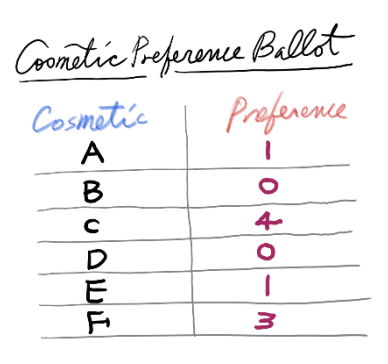시간 제한메모리 제한제출정답맞힌 사람정답 비율
2 초 512 MB3721058330.855%

## 문제

ICP(International Cosmetic Perfection) Company plans to survey the preferences of new m cosmetics in order to know which cosmetic is the most preferred. For this survey, ICPC selected n people as evaluators. Each evaluator must submit an ordered list of the preferences on those m cosmetics. Each evaluator can rank the cosmetics as follows:

• An evaluator can rank cosmetics with positive integer numbers, but the positive integers are not necessarily consecutive. The cosmetics with the smallest positive number are the most preferred ones, and the ones with the second smallest positive number are the second most preferred ones, and so on.
• An evaluator can give the same preference to more than one cosmetic. This indicates that this evaluator has no preference among those cosmetics.
• An evaluator may not rank some cosmetics. The unranked cosmetics are marked with number 0 in the ordered list. This indicates that this evaluator strictly prefers all ranked to all unranked ones, and has no preference among all unranked ones.For example, the right figure shows an ordered list of the preferences on six cosmetics A, B, C, D, E, F that an evaluator submitted. The cosmetics A, C, E, F are ranked with nonconsecutive integers of 1, 4, 1, 3, respectively. The other two cosmetics B and D are not ranked, thus they are marked with 0 . A and E have the same preference, which are the most preferred cosmetics because their preference number is the smallest positive integer. The unranked B and D are less preferred than the ranked ones. As a result, the preference order of this evaluator is A = E ≻ F ≻ C ≻ B = D, where X ≻ Y means that X is strictly preferred to Y and X = Y means the same preference.

Which cosmetics are the most preferred ones from n evaluators in this preference survey? We must now define which one is preferred to another. Let d(X, Y) be the number of evaluators who strictly prefer X to Y. A path from X to Y is a sequence of distinct cosmetics C1, ..., Ck such that C1 = X, Ck = Y, and d(Ct, Ct+1) > d(Ct+1, Ct) for every t = 1, ... , k − 1. The preference index of this path is defined as the minimum of d(Ct, Ct+1) for 1 ≤ t < k. For two distinct cosmetics X and Y that are connected by a path from X to Y, the preference strength S(X,Y) is the maximum preference index over all paths from X to Y. If there is no path from X to Y, then S(X,Y) is defined as zero. Cosmetic X is one of the most preferred cosmetics from this survey if and only if S(X,Y) ≥ S(Y,X) for every Y other than X. You note that it has been known for this type of surveys that there always exists at least one cosmetic that is preferred the most.

Given preference lists of n evaluators for m cosmetics, write a program to output all the most preferred cosmetics.

## 입력

Your program is to read from standard input. The input starts with a line containing two integers, m and n (1 ≤ m ≤ 500, 1 ≤ n ≤ 500), where m is the number of cosmetics and n is the number of evaluators. The cosmetics are numbered from 1 to m, and the evaluators are numbered from 1 to n. In the following n lines, the i-th line contains m nonnegative integers that represent the preference values for the m cosmetics of the evaluator i. The preference values are ordered from the cosmetic 1 to the cosmetic i. The zero values in the list mean that the evaluator unranked the corresponding cosmetics. The preference values are no more than 106.

## 출력

Your program is to write to standard output. Print exactly one line. The line should contain the numbers of the cosmetics that are preferred the most. Such cosmetic numbers must appear in increasing order.

## 예제 입력 1

3 4
1 1 1
0 0 0
2 2 2
3 3 3


## 예제 출력 1

1 2 3


## 예제 입력 2

4 5
1 0 1 1
1 1 5 2
2 1 3 6
0 1 0 1
1 2 2 2


## 예제 출력 2

1 2


## 예제 입력 3

5 4
0 1 0 2 1
1 7 2 1 0
4 5 2 3 3
1 2 9 0 2


## 예제 출력 3

5


## 출처

• 문제의 오타를 찾은 사람: khsoo01